This is a work in progress, please see the in-line comments for a description of what is going on here.

``````# load required libraries
library(soilDB)
library(aqp)
library(sharpshootR)
library(sf)
library(scales)

# sample data from soilDB packaeg
data("loafercreek")

# keep first 10 profiles
x <- loafercreek[1:10, ]

# default margins are too wide, make smaller
par(mar=c(1,1,1,1))

# profile sketches
plotSPC(x, label='pedon_id', id.style='side')``````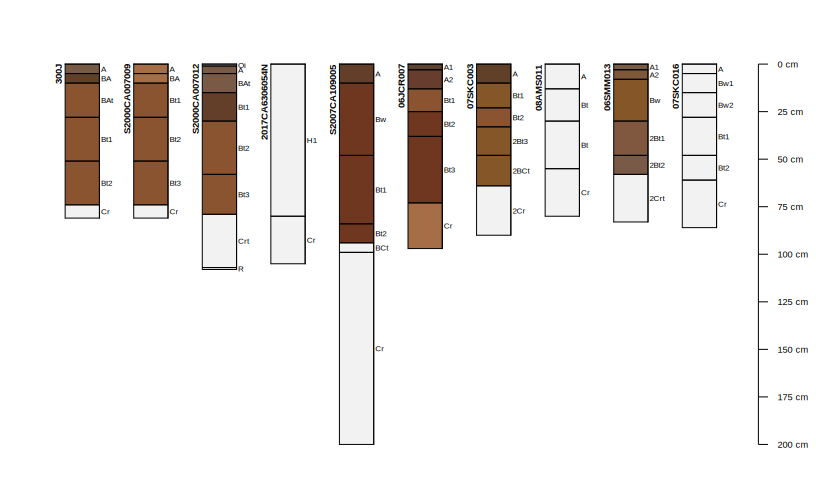``````# group profiles by hillslope position
groupedProfilePlot(x, 'hillslopeprof', label='pedon_id', id.style='side')``````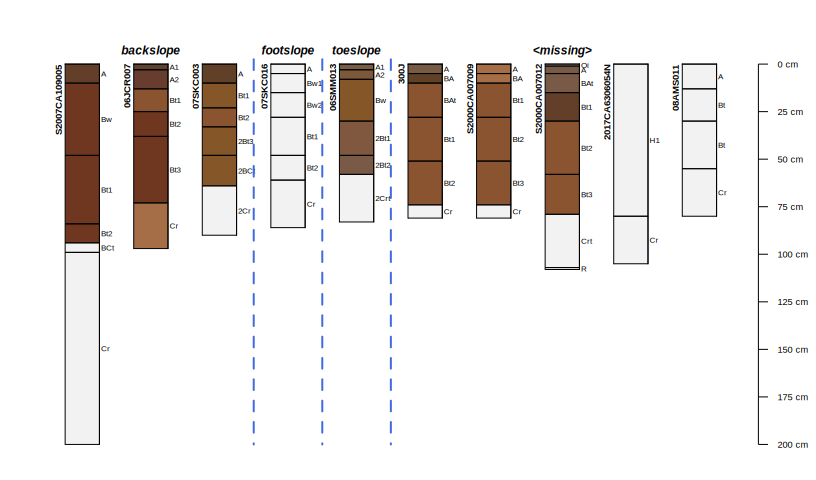``````# extract site data so that we can annotate wit PSCS depths
s <- site(x)

# reminder of ID used by SPC
idname(x)``````
``##  "peiid"``
``````# subset site data into ID and PSCS top / bottom
s <- s[, c('peiid', 'psctopdepth', 'pscbotdepth')]

names(s)[2:3] <- c('top', 'bottom') ``````
``````# plot sketches and annotate with PSCS
par(mar = c(1, 1, 1, 1))

# omit pedon IDs for now
plotSPC(x, print.id = TRUE, max.depth = 125, name.style = 'center-center', depth.axis = list(line = -3))

# add PSCS brackets as vertical var
addBracket(s, offset = -0.38, tick.length = 0, lwd = 10, col = rgb(red=0, green=0, blue=1, alpha=0.25))

# check structure of diagnostic horizon data
``````##    peiid           featkind featdept featdepb
## 1 115595    ochric epipedon        0       10
## 2 115595   argillic horizon       10       74
## 3 115595 paralithic contact       74       81
## 4 115819    ochric epipedon        0       10
## 5 115819   argillic horizon       10       74
## 6 115819 paralithic contact       74       81``````
``````# tabulate and sort
# sort(table(diagnostic_hz(x)\$featkind))

# add brackets for some diagnostic features
addDiagnosticBracket(x, offset = -0.38, kind='argillic horizon', col='red')
addDiagnosticBracket(x, offset = -0.38, kind='paralithic contact', col='black')``````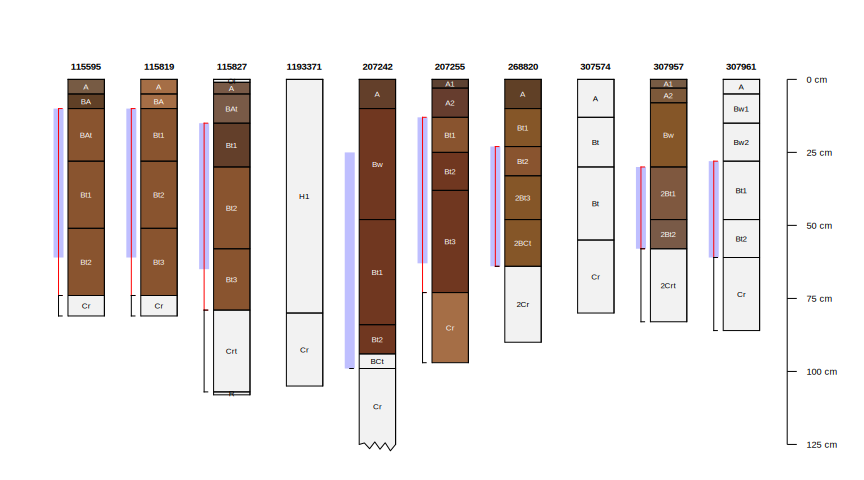Align profiles to an elevation gradient, regular spacing of sketches.

``````# experiment with re-ordering sketches
# order according to elevation as described in the field
new.order <- order(x\$elev_field)
new.order``````
``##    8  1  2  9 10  7  3  6  4  5``
``````# plot profiles in the new order
par(mar=c(4.5,1,1,1))
plotSPC(x, plot.order=new.order, print.id=TRUE)

# the "brackets" will automatically follow the new ordering
addBracket(s, tick.length = 0, lwd=10, col=rgb(red=0, green=0, blue=1, alpha=0.25))

# add an axis with elevation associated with each profile
axis(side=1, at = 1:length(x), labels = x\$elev_field[new.order], line = 1)
mtext('Elevation (m)', side = 1, line = 3.25)``````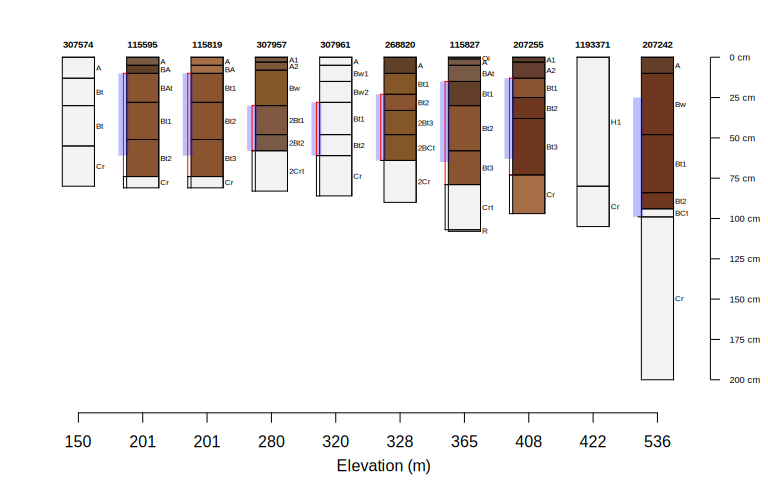Align profiles to an elevation gradient, relative spacing of sketches. Note that figure elements need to be explicitly ordered with `[new.order]`.

``````pos <- rescale(x\$elev_field, to = c(1, length(x)))
pos <- fixOverlap(pos, thresh = 0.65)

new.order <- order(x\$elev_field)

# plot profiles in the new order
par(mar=c(4.5,1,1,1))
plotSPC(x, plot.order = new.order, print.id = TRUE, relative.pos = pos[new.order], , max.depth = 125, name.style = 'center-center', depth.axis = list(line = -3))

# the "brackets" will automatically follow the new ordering
addBracket(s, offset = -0.33, tick.length = 0, lwd=8, col=rgb(red=0, green=0, blue=1, alpha=0.25))
addDiagnosticBracket(x, offset = -0.33, kind='argillic horizon', col='red')

# add an axis with elevation associated with each profile
axis(side=1, at = pos[new.order], labels = x\$elev_field[new.order], line = 1)
mtext('Elevation (m) | Not to Scale', side = 1, line = 3.25)``````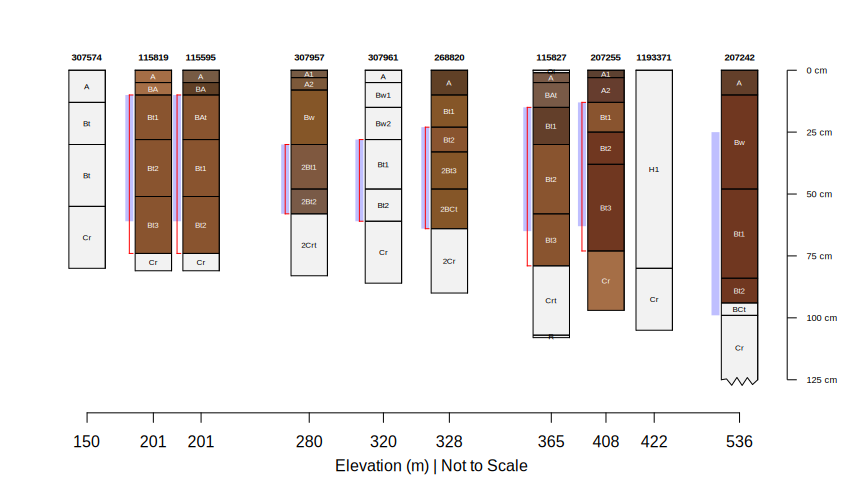Test out some new features in `aqp`.

``````par(mar=c(4.5,1,3,1))
plotSPC(x, color='total_frags_pct', plot.order=new.order, print.id=FALSE, max.depth = 125, name.style = 'center-center', depth.axis = list(line = -3))

# the "brackets" will automatically follow the new ordering
addBracket(s, offset = -0.38, tick.length = 0, lwd=10, col=rgb(red=0, green=0, blue=1, alpha=0.25))
addDiagnosticBracket(x, offset = -0.38, kind='argillic horizon', col='red')
addDiagnosticBracket(x, offset = -0.38, kind='paralithic contact', col='black')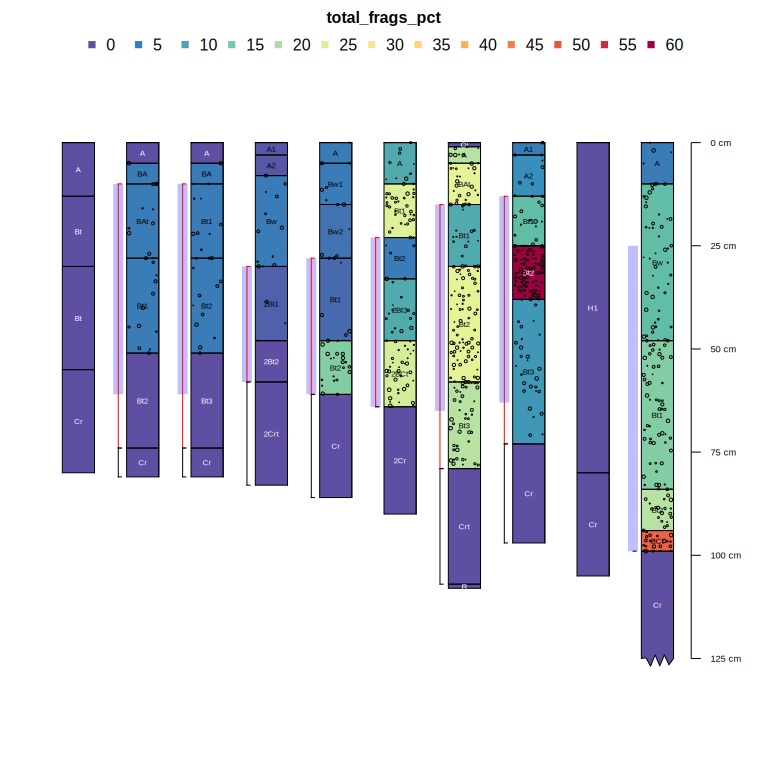``````plotSPC(x, color='total_frags_pct', plot.order=new.order, print.id=FALSE, relative.pos=jitter(1:length(x)), max.depth = 125, name.style = 'center-center', depth.axis = list(line = -3))